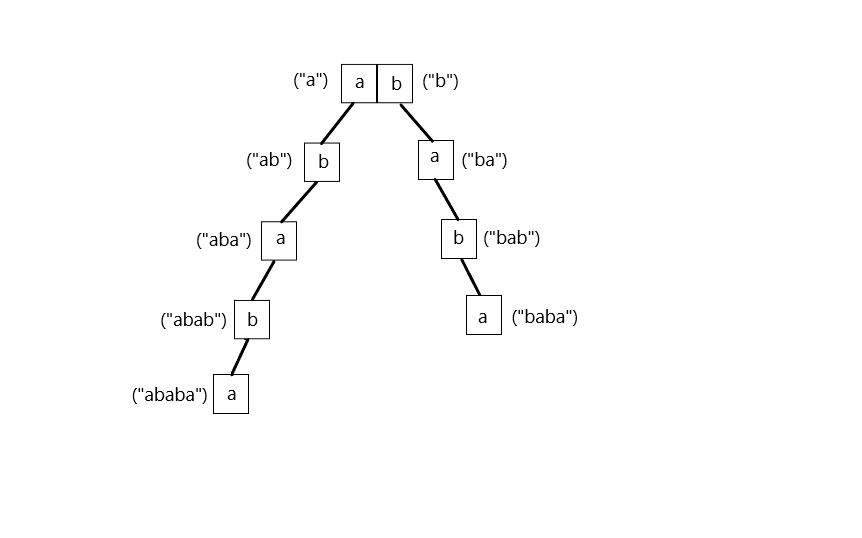# Number of Distinct Substrings in a String Using Trie

Problem Statement: Given a string of alphabetic characters. Return the count of distinct substrings of the string(including the empty string) using the Trie data structure.

Examples:

```Example 1:
Input:
S1= “ababa”
Output: Total number of distinct substrings : 10
Explanation: All the substrings of the string are a, ab, aba, abab, ababa, b, ba, bab, baba, a, ab, aba, b, ba, a. Many of the substrings are duplicated. The distinct substrings are a, ab, aba, abab, ababa, b, ba, bab, baba. Total Count of the distinct substrings is 9 + 1(for empty string) = 10.Example 2:
Input:
S1= “ccfdf”

Output: Total number of distinct substrings : 14
Explanation:
All the substrings of the string are c, cc, ccf, ccfd, ccfdf, c, cf, cfd, cfdf, f, fd, fdf, d, df, f. Many of the substrings are duplicated. The distinct substrings are c, cc, ccf, ccfd, ccfdf, cf, cfd, cfdf, f, fd, fdf, d, df. Total Count of the distinct substrings is 13 + 1(for empty string) = 14.
```

### Solution

Disclaimer: Don’t jump directly to the solution, try it out yourself first.

Intuition:

The basic intuition is to generate all the substrings and store them in the trie along with its creation. If a substring already exists in the trie then we can ignore, else we can make an entry and increase the count. Let’s see a detailed explanation.

Approach:

A typical node in a TRIE data structure looks like this:

• The 1st step is to create the trie structure and it generally consists of an array of nodes. Since we are going to have only alphabetic characters in the string, the maximum size of the array will be 26. We can also extend to accommodate upper case letters, if we want.
• Then, we are going to generate all substrings of the given string. It can be done by 2 nested loops. For each iteration, the outer loop fixes the starting point and the inner loop traverses the substring from the starting point to the end of the string.
• For each character encountered in the traversal of the inner loop, we are checking whether that particular node in the trie already contains the character or not.
• If it has, it means that the currently generated substring is a duplicate one. And we can just go to the next iteration to check the next character. But if the current character is not in the current node, then it means that the current substring generated is a brand new one.
• And we are creating a new node for the latest character. Since it is a new substring, we are increasing the total count.
• Since, the problem also demands to include empty string, we can just add 1(for empty string) to the total counts we got earlier..

Code:

## C++ Code

``````#include<iostream>

using namespace std;

struct Node {

bool containsKey(char ch) {
return (links[ch - 'a'] != NULL);
}

Node * get(char ch) {
}

void put(char ch, Node * node) {
}
};

int countDistinctSubstrings(string & s) {
Node * root = new Node();
int count = 0;
int n = s.size();
for (int i = 0; i < n; i++) {
Node * node = root;

for (int j = i; j < n; j++) {
if (!node -> containsKey(s[j])) {
node -> put(s[j], new Node());
count++;
}
node = node -> get(s[j]);
}

}
return count + 1;
}

int main() {
string s1 = "ababa";
cout << "Total number of distinct substrings : " << countDistinctSubstrings(s1);
cout << "\n";

string s2 = "ccfdf";
cout << "Total number of distinct substrings : " << countDistinctSubstrings(s2);

return 0;
}``````

Output:

Total number of distinct substrings: 10
Total number of distinct substrings: 14

Time Complexity: O(n2), because we have to go through the entire string for all possible different starting points in order to generate all the substrings.

Space Complexity: O(n2), because in the worst case, all the substrings can be distinct and there will be a node for every substring. Number of substrings of a string of length n is (n * (n + 1) / 2). Hence in the worst case, space complexity will be O(n2).

## Java Code

``````import java.util.*;

public class Solution {
public static void main(String[] args) {

String s1 = "ababa";
System.out.println("Total number of distinct substrings : " +
countDistinctSubstrings(s1));

String s2 = "ccfdf";
System.out.println("Total number of distinct substrings : " +
countDistinctSubstrings(s2));

}

public static int countDistinctSubstrings(String s) {
Node root = new Node();
int n = s.length();
int count = 0;

for (int i = 0; i < n; i++) {
Node node = root;

for (int j = i; j < n; j++) {
if (!node.containsKey(s.charAt(j))) {
node.put(s.charAt(j), new Node());
count++;
}
node = node.get(s.charAt(j));
}
}
return count + 1;
}
}

class Node {

boolean containsKey(char ch) {
return (links[ch - 'a'] != null);
}

Node get(char ch) {
}

void put(char ch, Node node) {